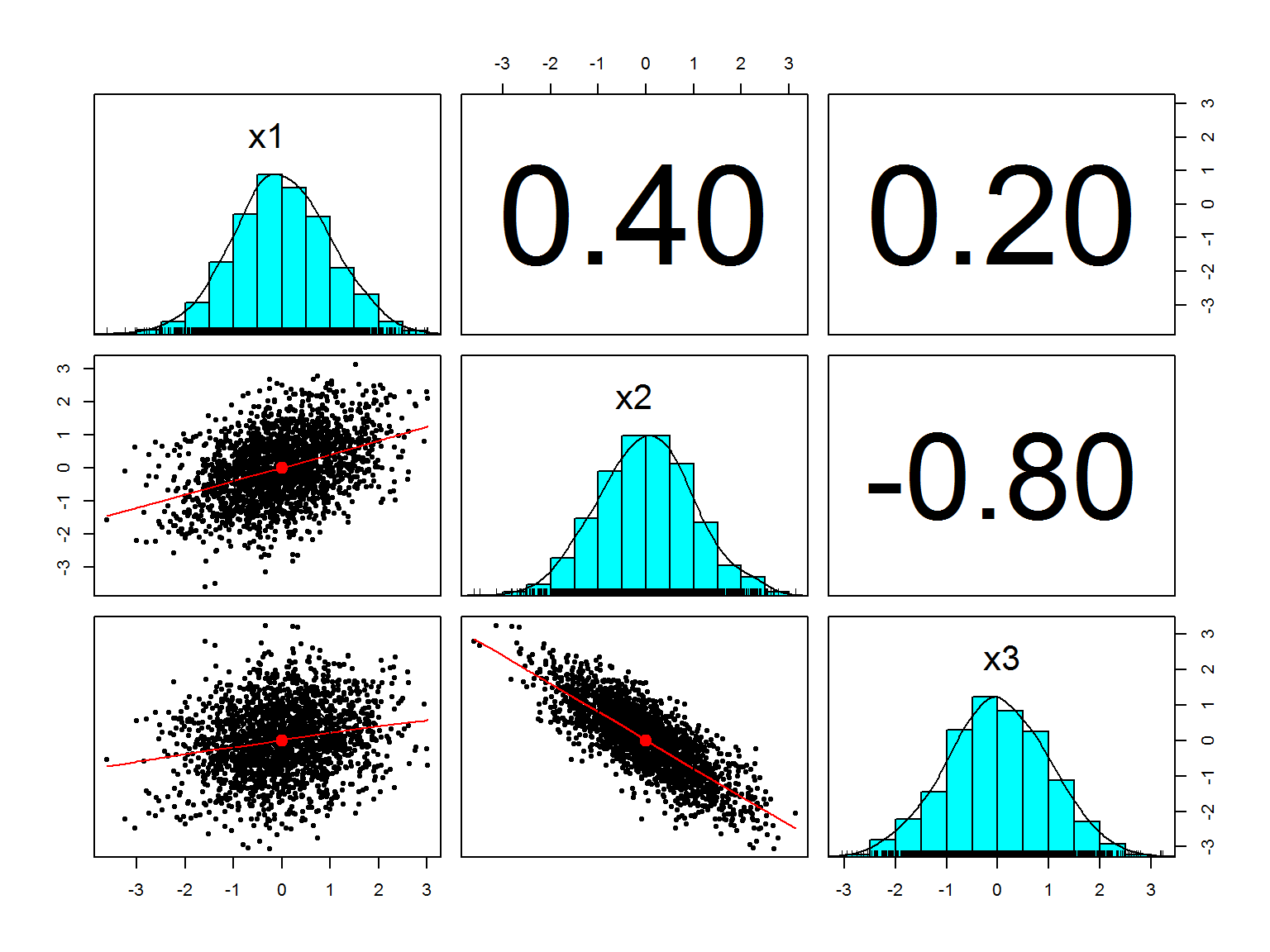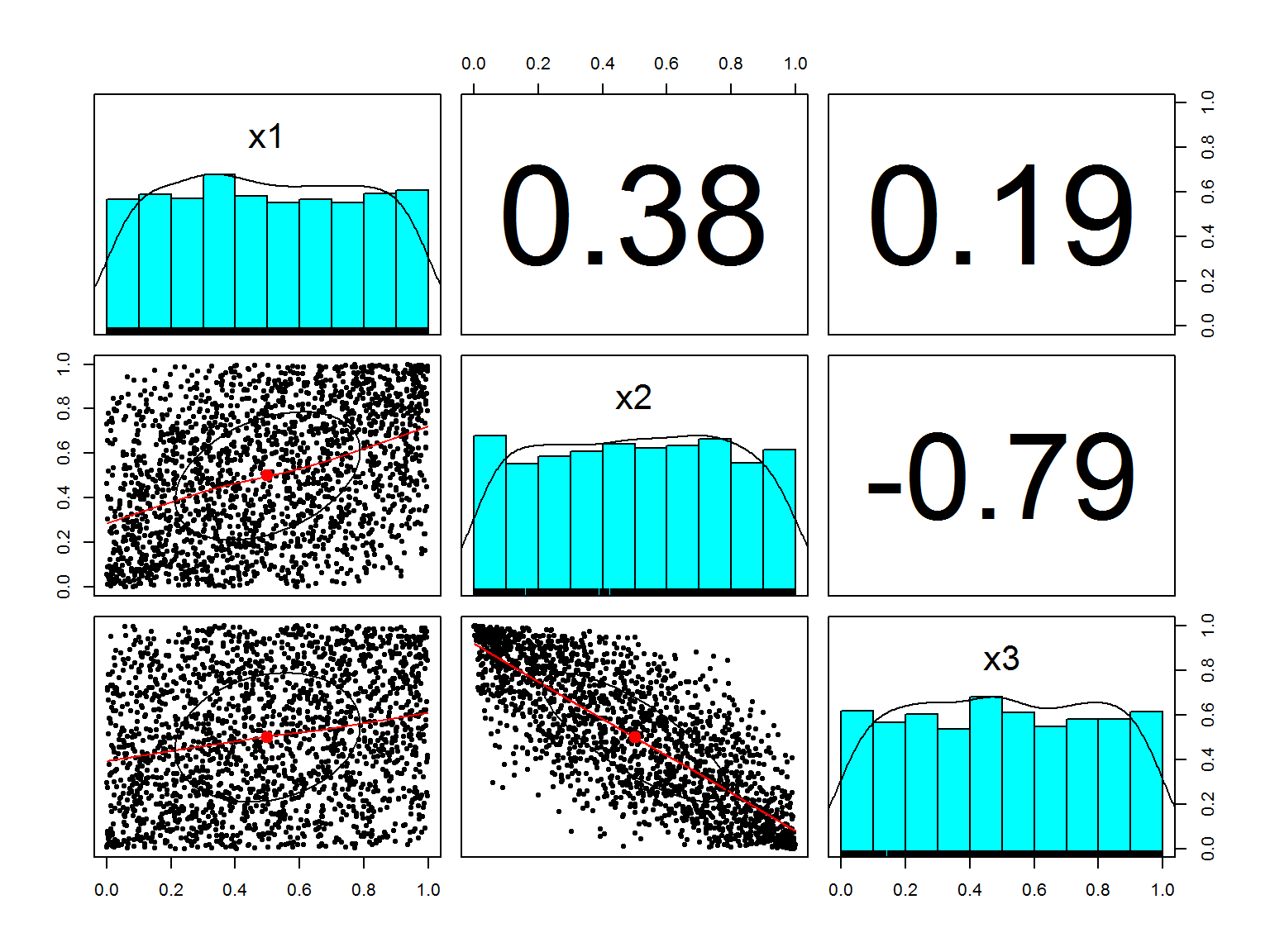Refs:

## Introduction

library(MASS)  # use: mvrnorm
library(psych) # use: pairs.panels

set.seed(100)

The next code creates a sample from the given multivariate normal distribution:

m <- 3
n <- 2000
mu    <- rep(0, m)
sigma <- matrix(c(1.0,  0.4,  0.2,
0.4,  1.0, -0.8,
0.2, -0.8,  1.0), nrow=3)

x <- mvrnorm(n, mu=mu, Sigma=sigma, empirical=TRUE)
colnames(x) <- paste0("x",1:m)
cor(x, method='spearman')  # check sample correlation
##           x1         x2         x3
## x1 1.0000000  0.3812244  0.1937548
## x2 0.3812244  1.0000000 -0.7890814
## x3 0.1937548 -0.7890814  1.0000000

Function mvrnorm is nice to generate correlated random variables but there is a restriction, the marginal functions are normal distributions:

pairs.panels(x)Many empirical evidence have skewed or heavy tailed marginals. What if we would like to create a similar correlated random variables but with arbitrary marginals, say, a Gamma(2,1), a Beta(2,2), and a t($$\nu$$=5) distribution?

Here’s a possible algorithm to do that:

1. Generate the variables $$x_i$$ from a Gaussian multivariate (as we did)

2. Remembering the probability integral transformation:

$X \sim F_X \Rightarrow U = F_X(X) \sim \mathcal{U}(0,1)$

transform $$x_i$$ using the Gaussian cdf, $$\Phi$$ (in R is called pnorm), $$u_i = \Phi(x_i)$$, where $$u_i$$ have marginal uniform distributions but are still correlated as variables $$x_i$$.

u <- pnorm(x)
pairs.panels(u)plot3d(u[,1],u[,2],u[,3],pch=20,col='navyblue')